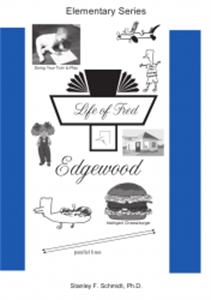# Life of Fred Edgewood

• \$ 15.00
Sold Out! - Semester Rental

Semester rental: 5 month period, beginning any time of the year. Just let us know in the checkout notes what month you would like to start your rental.

The fifth book in the Life of Fred Elementary Series, Edgewood; the first book of second-grade material.

Math Concepts

• Concurrent Lines
• Parallel Lines
• Trapezoids
• Right Angles
• Functions
• Rhombuses
• Constant Functions
• Median Average
• Writing Larger Numbers with Commas
• Using Logarithms to Solve 2x = 5
• Birdie Rule for Logarithms
• Finding Approximate values for log 5 on a Calculator
• Bar Graphs
• < (less than)
• Numbers that Add to 8
• 10 and 12
• Paralellograms
• Rows and Columns of a Matrix
• Quarter of an Hour
• Half Dozen
• Six Examples of Functions: Add, Subtract, Multiply, Divide, Tangent, and Derivative
• and more.